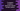# Write a C program to draw spiral two column number pattern# Introduction:

In this C programming tutorial, we will learn how to draw a spiral two column number pattern. This pattern looks like below:

You can see that the numbers in the pattern are continuous but in spiral order. This is a beginner level C program and with it, you will get the basic understanding of reading and writing user inputs, using for loop and using if-else condition in a C program.

Our program will ask the user to enter the height of the pattern. Based on the entered height, it will print out the result.

Before writing the code, let me quickly show you the algorithm that we will use in this program:

## Algorithm:

1. Take the height of the pattern from the user.
2. Run one loop to print the numbers for that height.
3. Print two numbers at a time inside the loop.
4. Exit the loop after all numbers are printed in the spiral order.

### C program:

We are using the same steps of the above algorithm in the C program. Here is it:

``````#include<stdio.h>

int main()
{
//1
int height, i;
int flag = 0;

//2
printf("Enter the height :");
scanf("%d", &height);

//3
for (i = 0; i < height*2; i += 2)
{
//4
if (flag == 0)
{
printf("%d %d\n", (i + 1), (i + 2));
flag = 1;
}
else
{
//5
printf("%d %d\n", (i + 2), (i + 1));
flag = 0;
}
}

return 0;
}
``````

### Explanation:

The commented numbers in the above program denote the step numbers below:

1. Create three integer variables : “height”, “i” and “flag”. The “height” variable is for storing the height of the pattern once the user will enter a value, “i” is for using in a loop and “flag” is to use as a marker. I will explain below what this “flag” is for.

2. Ask the user to enter the height of the pattern. Read it and store it in the “height” variable. We are using printf to write the message to the user and scanf to read the value.

3. Run one for loop to print the values. Notice that the loop will run from i = 0 to i = height * 2 and on each iteration of the loop, the value of i will increment by 2. i.e. for the first iteration, its value is 0, 2 for the second iteration, 4 for the third etc.

As it is incrementing by 2 on each iteration, we are running this loop upto height * 2.

1. Inside the loop, we are printing two numbers at a time. e.g. for the first iteration, we will print 1 2, for the second iteration 4 3 etc. Here, we are checking the value of the flag variable. If it is 0, we are running the if block and setting its value to 1 and if its value is 1, we are running the else block.The first iteration will run the if block, second iteration will run the else block, third will if block again etc.

2. Check the values we are printing in the if and the else block carefully. We are printing these values based on the value of i.For the first iteration, i is 0, flag is 0 and we are printing 1 2 i.e. _ (i+1) (i+2)_.

For the second iteration, i is 2, flag is 1 and we are printing 4 3 i.e. _ (i+2) (i+1). For the third iteration, i is 4, flag is 0 and we are printing 5 6 i.e. _ (i+1) (i+2). etc.

### Sample Output:

``````Enter the height :12
1 2
4 3
5 6
8 7
9 10
12 11
13 14
16 15
17 18
20 19
21 22
24 23
``````

### Conclusion:

Using this approach, you can print a spiral pattern of any height you want. Try to run the above program and drop one comment below if you have any queries.